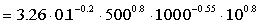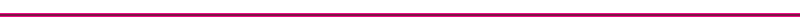Instantaneous Heat Transfer Coefficients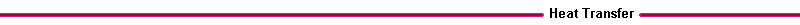A correlation used to compute the space averaged instantaneous heat transfer coefficient (Woschni, 1967 , "A universally applicable equation for the instantaneous heat transfer coefficient in the internal combustion engine", SAE Paper 670931): is: Nusselt # = 0.035 (Reynolds #)0.80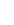Where: b = cylinder characteristic length (m), usually chosen to be the cylinder bore. k = gas thermal conductivity, (W/mK), typical value 0.06 n = gas kinematic viscosity, (m2/s), typical value 100 x 10-6 U = average gas velocity (m/s) During intake, compression, and exhaust, the average gas velocity U is proportional to the mean piston speed, Upiston. For intake and exhaust, U = 6.18 Upiston For compression, U = 2.28 Upiston The instantaneous heat transfer coefficient during combustion depends on the gas speed and cylinder pressure, which change significantly during the combustion process. During combustion and expansion, the gas speed U (m/s) induced by combustion is given by the following equation (Woschni, 1967) :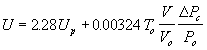Where To, Vo, and Po are reference intake cylinder temperature (K), volume (m3), and pressure (Pa), V is the instantaneous cylinder volume (m3), and DPc is the instantaneous pressure rise due to combustion relative to the unburned gas pressure at the same crankshaft angle. The unburned gas cylinder pressure is determined from thermodynamic analysis. An example pressure computation is given in the Cylinder Pressure page. For engines with significant swirl, the gas velocities are higher. The gas properties in the correlation equation are evaluated at the instanteous average cylinder temperature determined from the ideal gas law: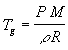Where: P = instantaneous cylinder pressure (kPa) r = instantaneous mixture density, mass/volume (kg/m3) M = averaged molecular weight (kg/kmol) R = universal gas constant, 8.314 kJ/kmol K If P= 500 kPa, r = 5 kg/m3, M = 40 kg/kmol,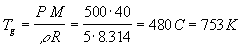The thermal conductivity k varies as  T^3/4 , and the viscosity varies as T^0.62 , so that the instantaneous heat transfer coefficient can be written as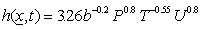If b=0.1m, P = 500 kPa, T = 1000 K, and U = 10 m/s,